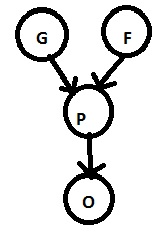# Needs and Examples of Probabilistic graphical model (PGMs) | Machine Learning

In this article, we are going to learn about the needs and examples of PGMs (Probabilistic graphical model).
Submitted by Bharti Parmar, on March 13, 2019

## Needs of PGMs

We have many algorithms for working with graphs and statistics. Using PGMs we can simulate dynamics even of a nuclear power plant, create models of chemical components and many other things. So, the probabilistic graphical model is very rich frameworks for encoding probabilistic distributions aver complex domains.

1. Compact graphical representation: It is a PGM framework used to create and represent compact graphical models of complex real-world scenarios.
2. Intuitive diagram of complex relationships: PGM give us an intuitive diagram of the complex relationship between stochastic variables.
3. Convenient from computational aspects: PGM also convenient from the computational point of view, since we already have algorithms for working with graphics and statistics.
4. Dynamic simulation of models: Using PGM we can simulate dynamics of industrial establishments, create models and many other things.

## Example of PGMs

• We have an image and we have to predict it contains a dog or cat in the image or we have an image of handwritten characters where we have to predict any 0-9 digit and A-Z character. Then, here, we use PGMs algorithm.
• We have a sentence like: "I am using PGM algorithm". So, here we have to use PGMs algorithm to predict this sentence; so, here learning could be part-of-speech (noun, pronoun, verb, adjective). This is important for a lot of difficult tasks such that: translate one language to another, text to speech.
• We can take an example of badminton to understand the concept of PGM: Consider, we have 4 binaries (yes/No) variable. Binary has two possible outcomes YES or NO.
1. Spot in the Olympic (yes/no)
2. Performance in the previous game (yes/no)
3. Good genetics (yes/no)
4. Good practice (yes/no)

DIA.:Now, the coach has to look out the two ways for the Olympics:

1. We have to use what we know about the relationships between variables to get information about more other difficult relationships.
2. Try to store our data and probability in a form where it is easy to input evidence and get out updated information.

### Components of graphical models

Nodes = Random variables and Edges = inter nodal dependencies

Sometimes, all the variables are available for observation and sometimes for subsets.

Conclusion: In this article, we have learnt about the needs of PGMs, the example of PGMs and components of the graphical model. Have a nice day! Happy Learning!

What's New

Top Interview Coding Problems/Challenges!

Languages: » C » C++ » C++ STL » Java » Data Structure » C#.Net » Android » Kotlin » SQL
Web Technologies: » PHP » Python » JavaScript » CSS » Ajax » Node.js » Web programming/HTML
Solved programs: » C » C++ » DS » Java » C#
Aptitude que. & ans.: » C » C++ » Java » DBMS
Interview que. & ans.: » C » Embedded C » Java » SEO » HR
CS Subjects: » CS Basics » O.S. » Networks » DBMS » Embedded Systems » Cloud Computing
» Machine learning » CS Organizations » Linux » DOS
More: » Articles » Puzzles » News/Updates